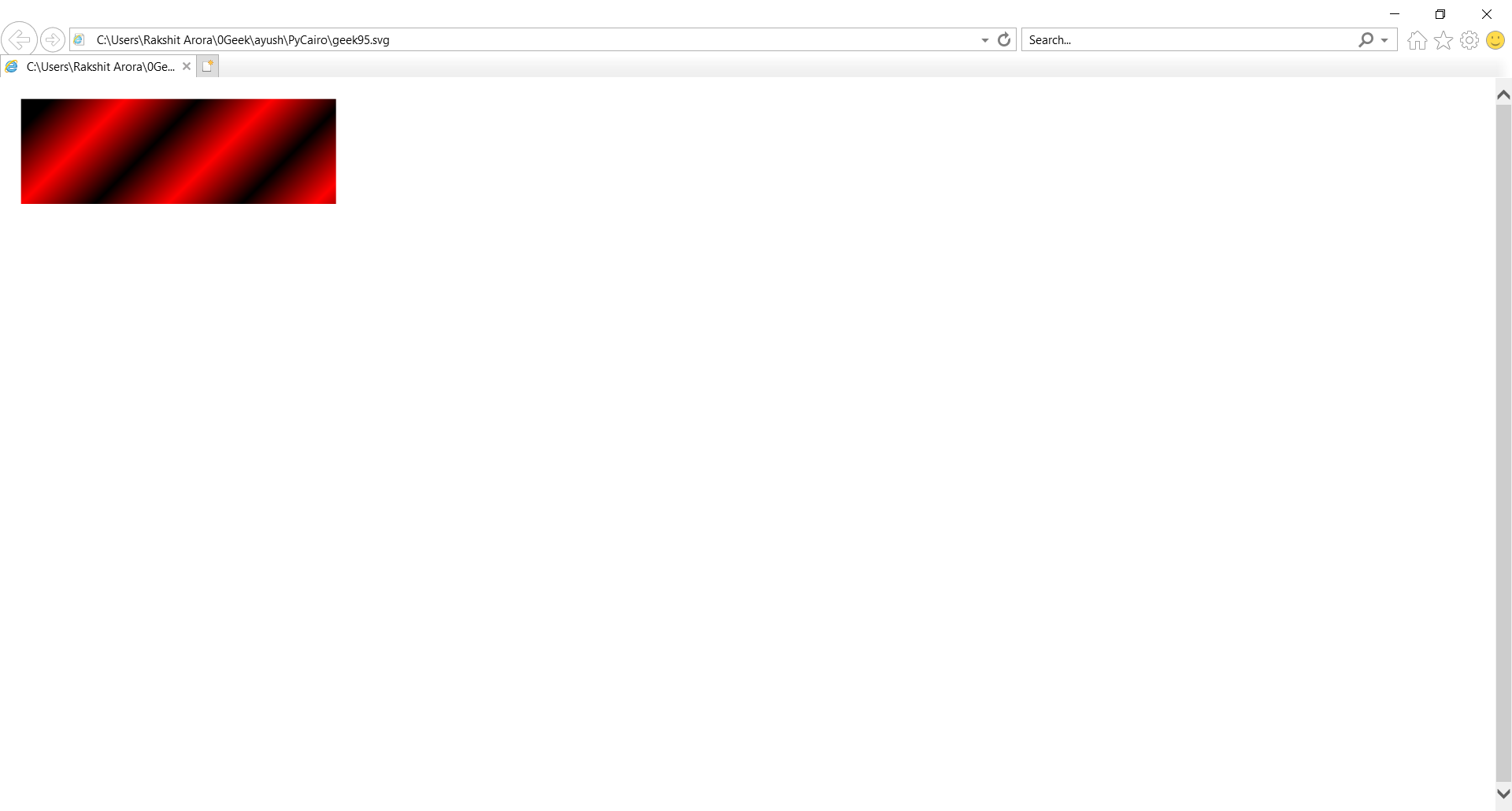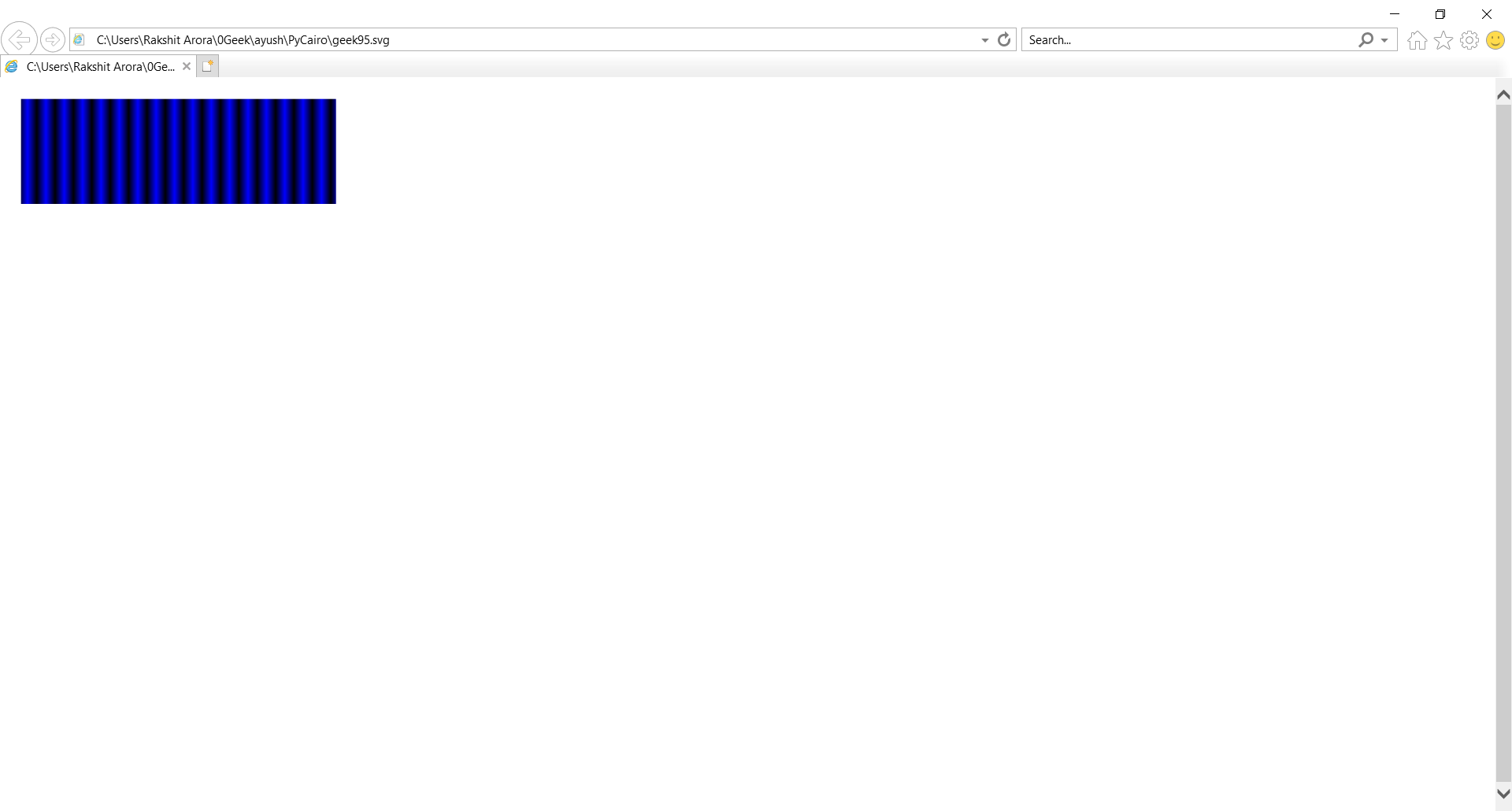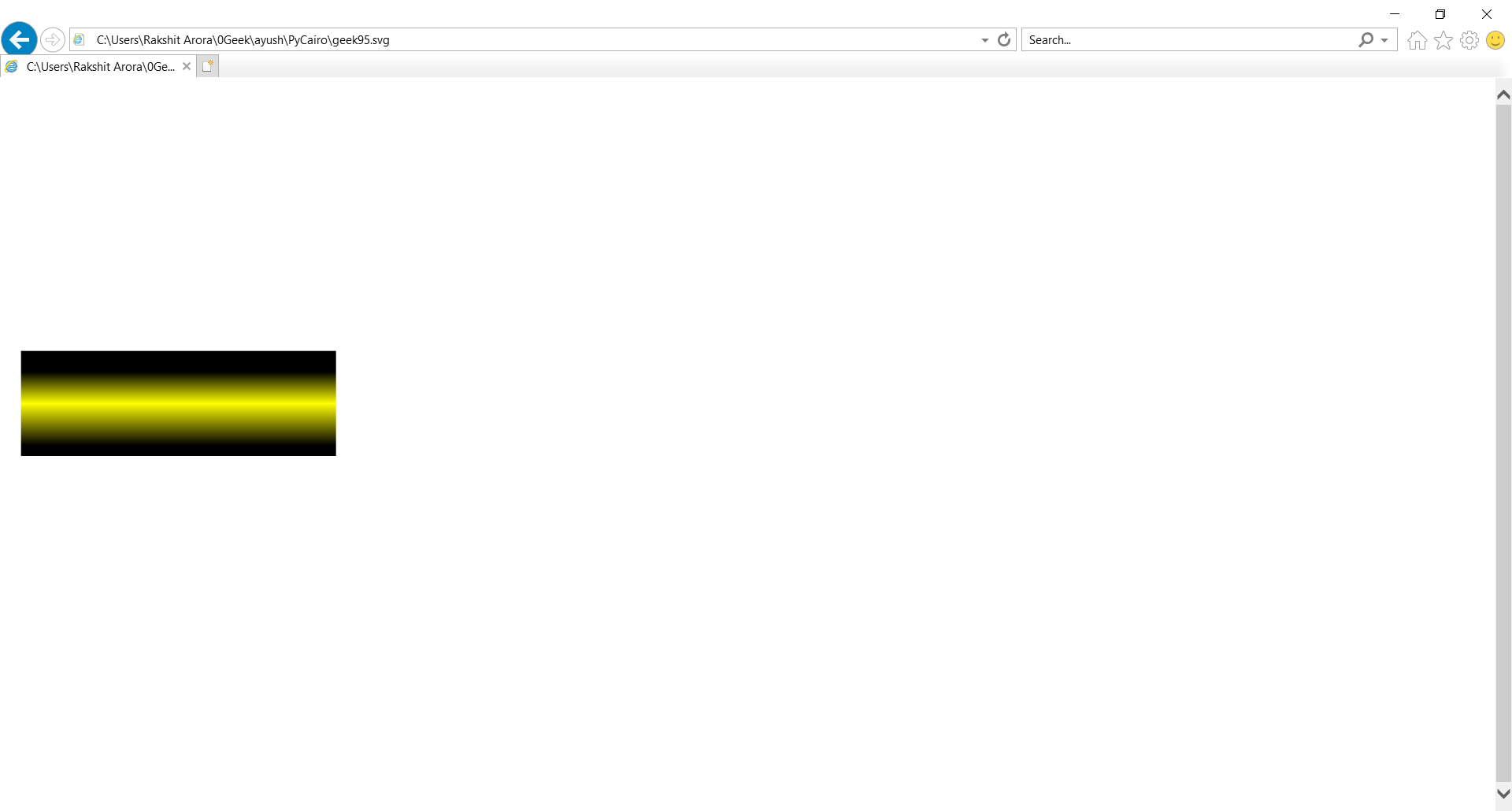GeeksforGeeks App
Open AppBrowser
Continue

## Related Articles

In the article we will learn to draw Liner gradients by python using PyCairo.  Pycairo is a Python module providing bindings for the cairo graphics library. This library is used for creating SVG i.e vector files in python. The easiest and quickest way to open an SVG file to view it (read only) is with a modern web browser like Chrome, Firefox, Edge, or Internet Explorer—nearly all of them should provide some sort of rendering support for the SVG format. The gradient line is defined by the center of the box containing the gradient image and by an angle. The colors of the gradient are determined by two or more points: the starting point, the ending point, and, in between, optional color-stop points.

Linear gradients are blendings of colours or shades of colours along a line. They are represented by a cairo.LinearGradient class in PyCairo.

Steps of Implementation :

1. Import the PyCairo module.
2. Create a SVG surface object and add context to it.
3. Creating a liner gradient object.
4. Defining loops or Condition, for adding color stripes
5. Creating a shape
6. The source is used to fill the interior of a rectangle by calling the fill ( ) method.

Example 1 :

## Python

 `# importing pycairo``import` `cairo` `# creating a SVG surface``# here geek95 is file name & 700, 700 is dimension``with cairo.SVGSurface(``"geek95.svg"``, ``700``, ``700``) as surface:` `    ``# creating a cairo context object for SVG surface``    ``# using Context method``    ``context ``=` `cairo.Context(surface)` `    ``# Creating a liner gradient object.``    ``lg1 ``=` `cairo.LinearGradient(``0.0``, ``0.0``, ``350.0``, ``350.0``)` `    ``# Creating Loops for adding color stripes``    ``count ``=` `1``    ``i ``=` `0.1``    ``while` `i < ``1.0``:``        ``if` `count ``%` `2``:``            ``lg1.add_color_stop_rgba(i, ``0``, ``0``, ``0``, ``1``)``        ``else``:``            ``lg1.add_color_stop_rgba(i, ``1``, ``0``, ``0``, ``1``)``        ``i ``=` `i ``+` `0.1``        ``count ``=` `count ``+` `1` `    ``# Creating Shape``    ``context.rectangle(``20``, ``20``, ``300``, ``100``)` `    ``#``    ``context.set_source(lg1)``    ``# Fill the color inside the rectangle``    ``context.fill()` `    ``# printing message when file is saved``print``(``"File Saved"``)`

Output :Example 2 :

## Python3

 `# code``import` `cairo``print``(``"GFG"``)``# importing pycairo` `# creating a SVG surface``# here geek95 is file name & 700, 700 is dimension``with cairo.SVGSurface(``"geek95.svg"``, ``700``, ``700``) as surface:` `    ``# creating a cairo context object for SVG surface``    ``# using Context method``    ``context ``=` `cairo.Context(surface)` `    ``# Creating a liner gradient object.``    ``lg2 ``=` `cairo.LinearGradient(``0.0``, ``0.0``, ``350.0``, ``0.0``)` `    ``count ``=` `1``    ``i ``=` `0.05``    ``# Creating Loops for adding color stripes``    ``while` `i < ``0.95``:``        ``if` `count ``%` `2``:``            ``lg2.add_color_stop_rgba(i, ``0``, ``0``, ``0``, ``1``)``        ``else``:``            ``lg2.add_color_stop_rgba(i, ``0``, ``0``, ``1``, ``1``)``        ``i ``=` `i ``+` `0.025``        ``count ``=` `count ``+` `1``    ``# Creating Shape``    ``context.rectangle(``20``, ``20``, ``300``, ``100``)``    ``context.set_source(lg2)``    ``# Fill the color inside the rectangle``    ``context.fill()``# printing message when file is saved``print``(``"File Saved"``)`

Output :Example 3 :

## Python3

 `# importing pycairo``import` `cairo` `# creating a SVG surface``# here geek95 is file name & 700, 700 is dimension``with cairo.SVGSurface(``"geek95.svg"``, ``700``, ``700``) as surface:` `    ``# creating a cairo context object for SVG surface``    ``# using Context method``    ``context ``=` `cairo.Context(surface)` `    ``# Creating a liner gradient object.``    ``lg3 ``=` `cairo.LinearGradient(``20.0``, ``260.0``,  ``20.0``, ``360.0``)` `    ``# adding color stripes``    ``lg3.add_color_stop_rgba(``0.2``, ``0``, ``0``, ``0``, ``1``)``    ``lg3.add_color_stop_rgba(``0.5``, ``1``, ``1``, ``0``, ``1``)``    ``lg3.add_color_stop_rgba(``0.9``, ``0``, ``0``, ``0``, ``1``)` `    ``# Creating Shape``    ``context.rectangle(``20``, ``260``, ``300``, ``100``)``    ``context.set_source(lg3)` `    ``# Fill the color inside the rectangle``    ``context.fill()` `# printing message when file is saved``print``(``"File Saved"``)`

Output :My Personal Notes arrow_drop_up